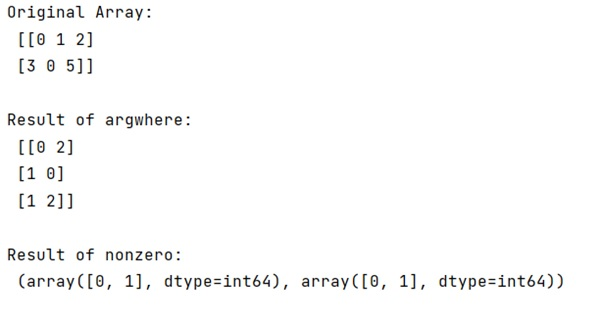# What's the difference between nonzero(a), where(a) and argwhere(a)?

Learn about the difference between nonzero(a), where(a) and argwhere(a) in Python.
Submitted by Pranit Sharma, on February 21, 2023

NumPy is an abbreviated form of Numerical Python. It is used for different types of scientific operations in python. Numpy is a vast library in python which is used for almost every kind of scientific or mathematical operation. It is itself an array which is a collection of various methods and functions for processing the arrays.

## Difference between nonzero(a), where(a) and argwhere(a)

Here, we'll learn the difference between nonzero(arr), where(arr), and argwhere(arr), and also we will understand when should we use each of them.

In NumPy, nonzero(arr), where(arr), and argwhere(arr), with arr being a numpy array, all seem to return the non-zero indices of the array but their working is different.

The numpy.argwhere(a) is almost the same as numpy.transpose(np.nonzero(a)), but produces a result of the correct shape for a 0D array.

The output of argwhere() is not suitable for indexing arrays and hence this is the reason why nonzero(arr) and where(arr) play an important role. The nonzero(arr) returns a tuple of arrays, one for each dimension of a, containing the indices of the non-zero elements in that dimension. The values in a are always tested and returned in row-major, C-style order.

As far as having both nonzero and argwhere(), they are conceptually different. nonzero is structured to return an object which can be used for indexing. This can be lighter-weight than creating an entire boolean mask if the 0's are sparse.

Let us understand with the help of an example,

## Python code to demonstrate the difference between nonzero(a), where(a) and argwhere(a)

```# Import numpy
import numpy as np

# Creating a numpy array
arr = np.array([[0,1,2],[3,0,5]])

# Display original array
print("Original Array:\n",arr,"\n")

# Using numpy.argwhere
res = np.argwhere(arr>1)

# Display result
print("Result:\n",res)
```

Output: